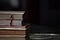Photo by Charl Folscher on Unsplash

# Non-interactive Zero-Knowledge Proof of Discrete Log Equality

I love zero-knowledge proofs (ZKPs), and I think we can build a new privacy-respecting world where we do not have to give away personal information. Normally within the interactive mode, Victor (the verifier) sends Peggy (the prover) a challenge (c), and Peggy sends back a proof. This can be improved with a non-interactive form and where Peggy can generate her own challenge and proof. This is known as a Non-interactive Zero-knowledge Proof (NIZK).

## Code

Here is the code for secp256k1 [here]:

`import randomimport hashlibfrom secp256k1 import curve,scalar_mult,point_add# Can we provex = random.randint(0, curve.n-1)k = random.randint(0, curve.n-1)r = random.randint(0, curve.n-1)G= curve.gM = scalar_mult(r,G)A = scalar_mult(k,G)B = scalar_mult(k,M)Y = scalar_mult(x,G)Z = scalar_mult(x,M)print (f"x={x}, k={k}\nG={G}\nM={M}\nA={A}\nB={B}\nY={Y}\nZ={Z}\n")G_x,_ = GM_x,_ = MA_x,_ = AB_x,y = BY_x,y = YZ_x,_ = Y# G,Y=xG, M , Z=xM, A=kG, B=kMc=hashlib.sha256(str(G_x+Y_x+M_x+Z_x+A_x+B_x).encode())digest = c.hexdigest()c = int(digest, 16)s=(k-c*x) % curve.nprint (f"c={c}, s={s}\n\n")A_ = point_add(scalar_mult(s,G), scalar_mult(c,Y))B_ = point_add(scalar_mult(s,M), scalar_mult(c,Z))A_x,y = A_B_x,y = B_c_=hashlib.sha256(str(G_x+Y_x+M_x+Z_x+A_x+B_x).encode())digest = c_.hexdigest()c_ = int(digest, 16)print (f"c={c_}")if (c==c_): print("\nProven!")else: print("\nNot proven!")print (f"\n\nA_x={A_x}, B_x={B_x}\n")`
`x=35416836453933982290692276802598715692905104517036468320404568776284340190568, k=61373966280138216830657815314248992751337679135921632607587699413140057084241G=(55066263022277343669578718895168534326250603453777594175500187360389116729240, 32670510020758816978083085130507043184471273380659243275938904335757337482424)M=(109313334810958477418321835497480696076356416408825979038916417641071785535510, 42436860500151098909615340702882320805111643294553141226977160459787210738143)A=(26826796251558955079235317283165928536253935451929951990183532315705741952623, 53202342644011362078490686414790012377384041579381932109021711369293617190511)B=(37944664236701417783091257402619603205531606235126016164345783936286432951278, 19113513177578213715672495528475763450271451179938795510108690299122769339603)Y=(106019702204816351426185591575742138944700259248555020992462182754709470335838, 26181941002749499427020424114275285554323295122454952021326958976654928222676)Z=(23511017161302932771891246196142291697198231493335006173532379876249399496914, 96295158920223023012699200915630000951625615202868968718354010341712645117480)c=14636018495143715303382353400769555713839065472325893247770338859312295689520, s=52676127977070219726246270185135606392788025498048831023138207679810143799338c=14636018495143715303382353400769555713839065472325893247770338859312295689520Proven!A_x=26826796251558955079235317283165928536253935451929951990183532315705741952623, B_x=37944664236701417783091257402619603205531606235126016164345783936286432951278`

# References

 Chaum, David, and Torben Pryds Pedersen. “Wallet databases with observers.” Annual international cryptology conference. Springer, Berlin, Heidelberg, 1992 [paper].

Professor of Cryptography. Serial innovator. Believer in fairness, justice & freedom. EU Citizen. Auld Reekie native. Old World Breaker. New World Creator.

## More from Prof Bill Buchanan OBE

Professor of Cryptography. Serial innovator. Believer in fairness, justice & freedom. EU Citizen. Auld Reekie native. Old World Breaker. New World Creator.